## Neural Networks for Face Recognition with TensorFlow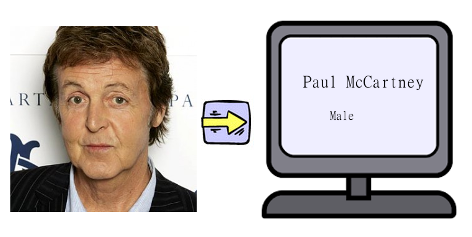In this assignment, you will learn to work with Google's TensorFlow framework to build a neural network-based face recognition system, and visualize the weights of the network that you train in order to obtain insights about how the network works. ### The input You will work with a subset of the [FaceSrub](http://vintage.winklerbros.net/facescrub.html) dataset (available under a [CC](https://creativecommons.org/licenses/by-nc-nd/3.0/) license). A subset of male actors is [here](subset_actors.txt) and a subset of female actors is [here](subset_actresses.txt). The dataset consists of URLs of images with faces, as well as the bounding boxes of the faces. The format of the bounding boxes is as follows (from the FaceScrub readme.txt file):
The format is x1,y1,x2,y2, where (x1,y1) is the coordinate of the top-left corner of the bounding box and (x2,y2) is that of the bottom-right corner, with (0,0) as the top-left corner of the image. Assuming the image is represented as a Python NumPy array I, a face in I can be obtained as I[y1:y2, x1:x2].
You may find it helpful to use a *modified* version of my script for downloading the image data. Note that get_data.py *will not* work "out of the box." You should work with the faces of the following actors:  acts= ['Gerard Butler', 'Daniel Radcliffe', 'Michael Vartan', 'Lorraine Bracco', 'Peri Gilpin', 'Angie Harmon'] Use the SHA-256 hashes provided with the dataset and exclude the images that you download whose hashes do not match the information in the text files. ### Handout code: Part A We are providing you with TensorFlow code to classify digits from the [MNIST](http://yann.lecun.com/exdb/mnist/) dataset [here](mnist_tf.py). The data that is read in by the code is available [here](mnist_all.mat). The initial weights are in [snapshot50.pkl](snapshot50.pkl). ### Part 0 Explore the MNIST digit dataset that the handout code classifies. In your report, include 10 images of each of the digits from the MNIST dataset, read in from mnist_all.mat. Run the code provided to you, and report on the performance of the digit classifier. ### Part 1 Describe the dataset of faces. In particular, provide at least three examples of the images in the dataset, as well as at least three examples of cropped out faces. Comment on the quality of the annotation of the dataset: are the bounding boxes accurate? Can the cropped out faces be aligned with each other? Explain why this is important in the context of classifying faces in the later parts of the assignment. ### Part 2 Build a face classifier to classify the faces of the six actors from acts. Modify the code provided to you to accomplish that. Use a fully-connected neural network with a single hidden layer. In your report, include the learning curves for the test, training, and validation sets, and the final classification performance on the the test, training, and validation sets. Include a description of your system. In particular, describe how you separated the data into training, text, and validation sets, preprocessed the input and initialized the weights, what activation function you used, and what the exact architecture of the network that you selected was. After selecting the training and validation sets, experiment with different settings to produce the best performance, and report what you did to obtain the best performance. You can start experimenting by rescaling all the faces to 32x32 using scipy.misc.imresize, converting them to grayscale, and scaling the images so that all the pixels are in the range \$$0..1\$$, so that each face is represented by \$$1024 = 32\times 32\$$ floats between \$$0\$$ and \$$1\$$. ### Part 3 We can visualize the weights of the networks that classify digits and faces in order to gain insight into how they work. For example, for a network with no hidden layer that classifies digits, we can reshape the weights that connect to the output that corresponds to the digit 3 into a \$$28\times 28\$$ image, and display it as a heatmap as follows: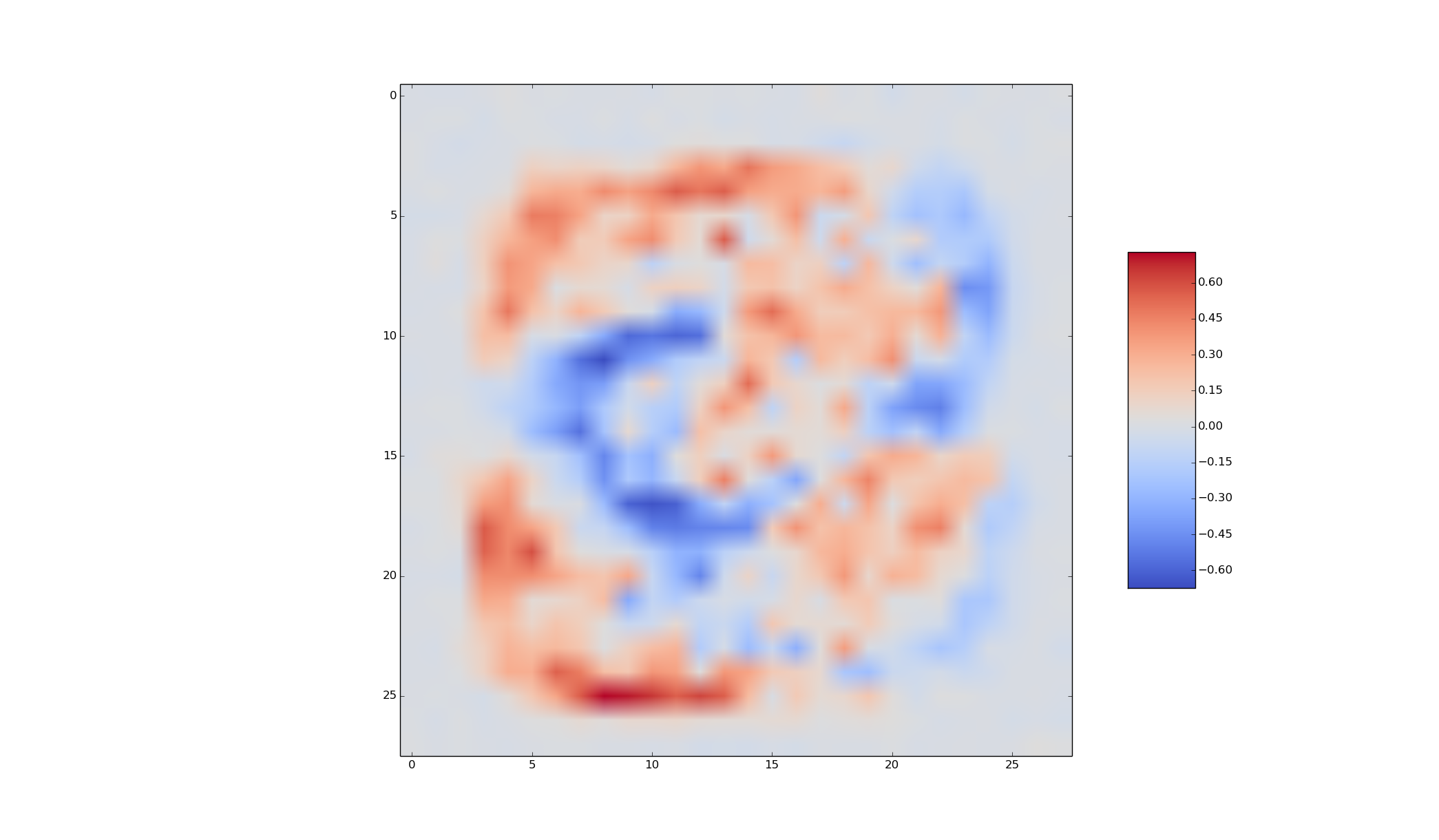Train a no-hidden-layer neural network (i.e., a multinomial logistic regression classifier) that classifies the actors' faces, and visualize the weights that correspond to each one of the six actors. Include the visualizations in your report. ### Part 4 (a) For a one-hidden-layer neural network, you can visualize the weights that connect the input layer to each hidden unit. For example, the weights of that connect the input layer to one of the hidden units can be visualized as follows: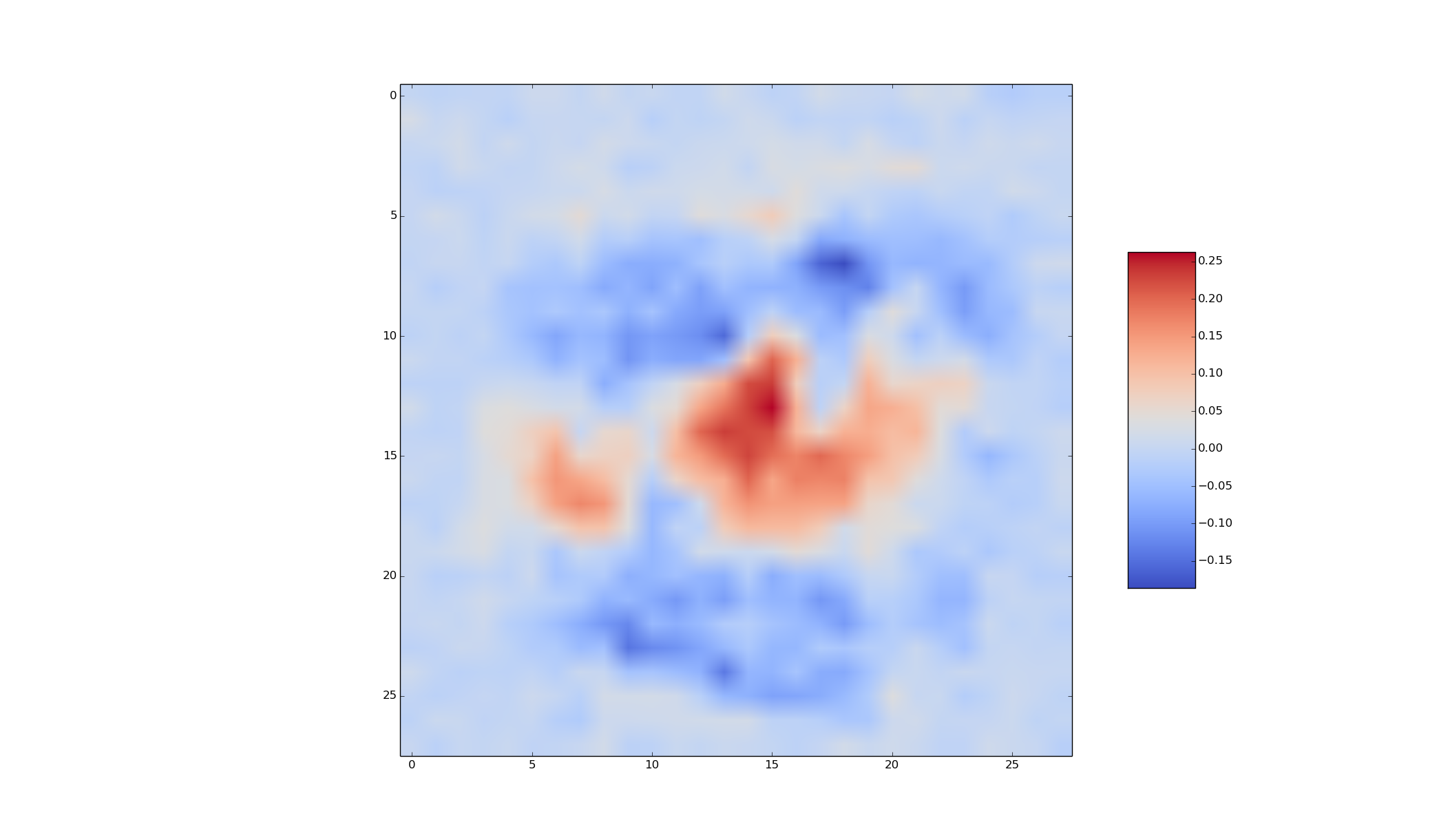The weights connecting the hidden unit visualized above to the output units that correspond to the digits \$$0..9\$$ are: [-0.17553222, 0.09433381, -0.75548565, 0.13704424, 0.17520368, -0.02166308, 0.15751396, -0.31243968, 0.12079471, 0.66215879]. Select two actors, and visualize the weights of the hidden units that you obtained that are useful for classifying input photos as those particular actors. Explain how you selected the hidden units (hint: one way to do that is to look at the weights connecting the hidden units to the output layer). Label your visualizations with the name of the actor, and display any other relevant information. A sample visualization is shown below.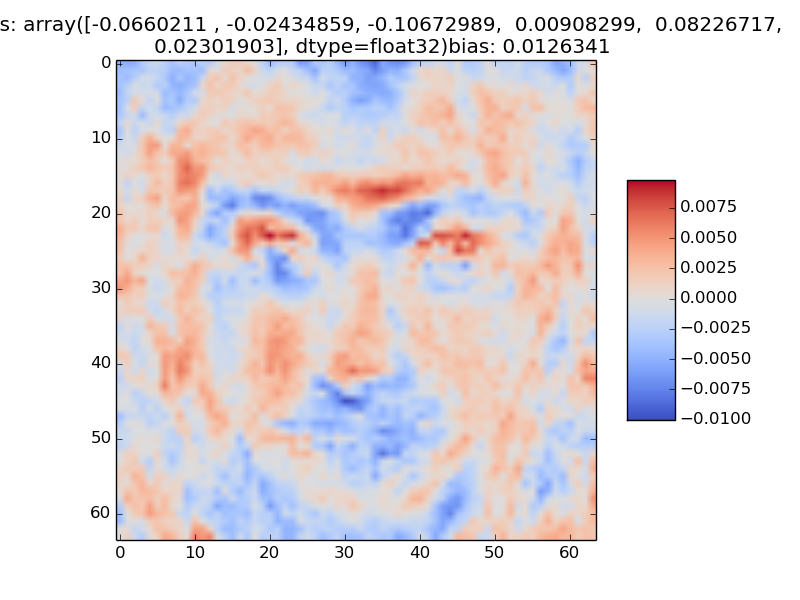Code to visualize the incoming weights to a particular neuron is below: # Code for displaying a feature from the weight matrix mW fig = figure(1) ax = fig.gca() heatmap = ax.imshow(mW[:,50].reshape((28,28)), cmap = cm.coolwarm) fig.colorbar(heatmap, shrink = 0.5, aspect=5) show() ### Part 4 (b) In class, we saw that L1 and L2 regularization can significantly affect the weights of the network, even if its effects on the classification performance are small. Experiment with using L2 regularization and modifying the size of the inputs in order to obtain visualizations that are similar in quality to the sample visualization. Report on what you found. ### Part 5 Increase the number of hidden units enough for significant overfitting to occur when you train the classifier. Visualize the weights that are associated with hidden units where the effect of overfitting is obvious. Report what you did to obtain those visualizations, and give an intuitive explanation for why you obtain the visualization that you do. ### Handout code: Part B We are providing the code for AlexNet [here](https://github.com/guerzh/tf_weights). ### Part 6 Extract the values of the activations of AlexNet on the face images. In your report, explain how you did that. Use those as features in order to perform face classification: train a fully-connected neural network that takes in the activations of the units in the AlexNet layer as inputs, and outputs the name of the person. In your report, include a description of the system you built and its performance, similarly to Part 3. It is possible to improve on the results of Part 3 by reducing the error rate by at least 30%. I recommend starting out with only using the conv4 activations. ### Part 7 For a specific photo of an actor, compute and visualize the gradient of the output of the network in Part 6 for that actor with respect to the input image. Visualize the positive parts of the gradient and the input image in two separate images. In your report, explain how exactly you obtained your visualization. Include the code that you used for this part in your report. Use tf.gradients. An example of a visualization of the gradient with respect to all three color channels is below, for a photo of Gerard Butler, is below.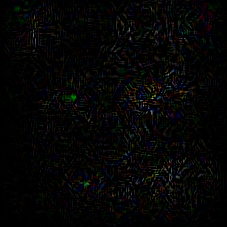Interpret the visualization that you obtain. ### Part 8 (bonus) Produce an interesting visualization of the hidden units that were trained on top of the AlexNet conv4 features. One possibility is to compute the Guided Backpropagation visualization, but you are encouraged to explore other possibilities.